SSAT Elementary Level Math : How to divide

Example Questions

1 2 48 49 50 51 52 53 54 56 Next →

Example Question #21 : Solve Multistep Word Problems Using The Four Operations: Ccss.Math.Content.4.Oa.A.3

Melissa is making holiday cookies. She madecookies and she's going to put them into small containers to give out to her friends. Each container holdscookies. How many containers can she fill completely?Explanation:

When we have a set of something that we are splitting up into groups, we divide. In this case, we are splitting up cookies into containers. We havecookies that we are dividing into containers. Each container holdscookies, so we dividebyto find out how many containers we can fill. We will letrepresent the number of containers that we can fill.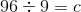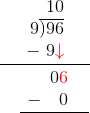We drop the remainder ofbecause the question asks how many containers we can fill completely.

Example Question #26 : The Number System

Use the computation shown to find the products: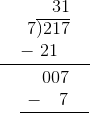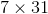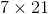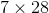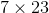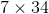Explanation:

The computation shows that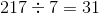with a remainder of.So it must be that: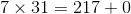Simplify.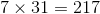The correct answer isExample Question #5 : Determine The Unknown Number In A Multiplication Or Division Equation: Ccss.Math.Content.3.Oa.A.4

Fill in the blank.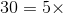________Explanation:

The opposite of multiplication is division. In order to find the missing piece of this multiplication problem we can divide.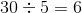So: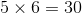1 2 48 49 50 51 52 53 54 56 Next →